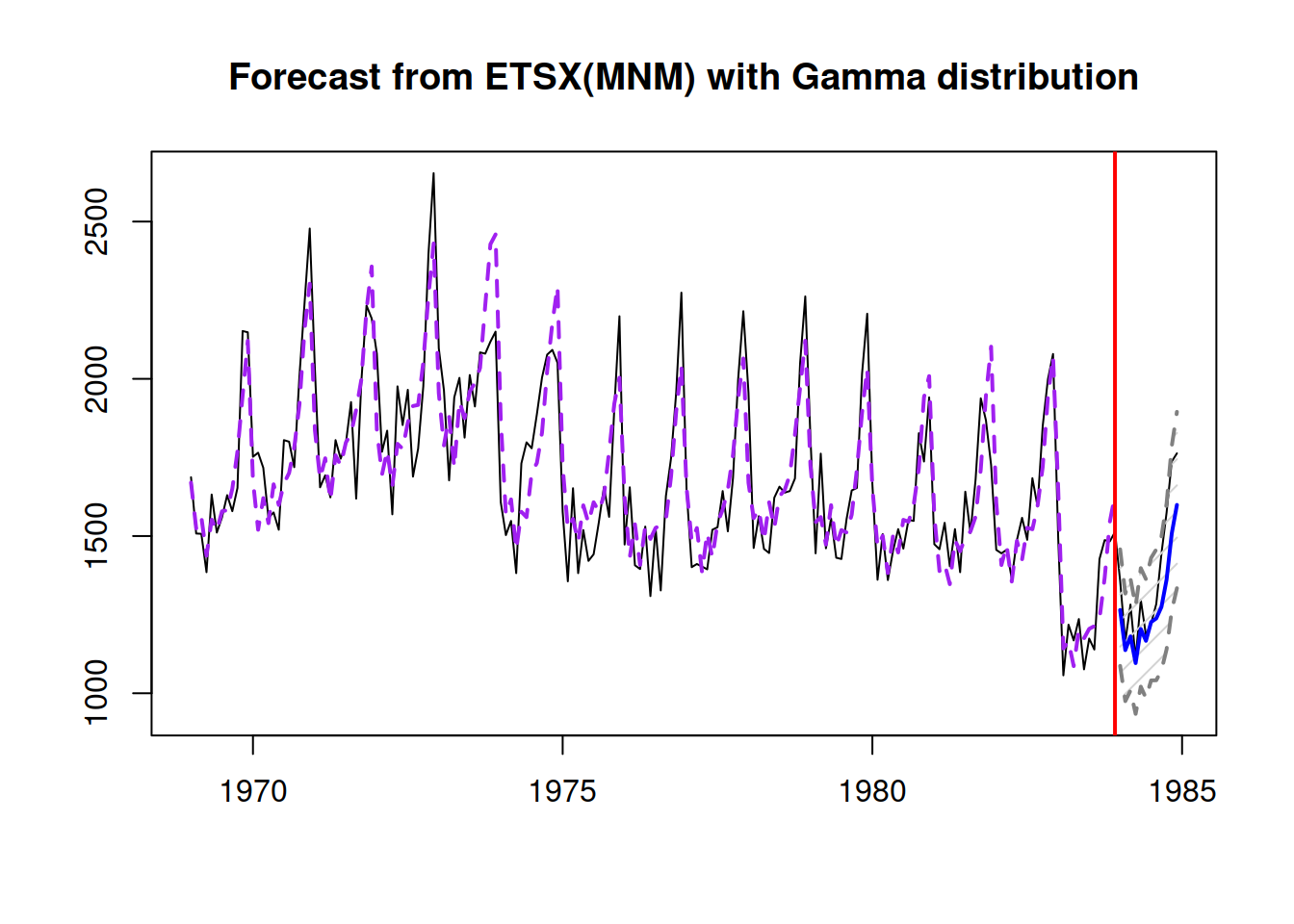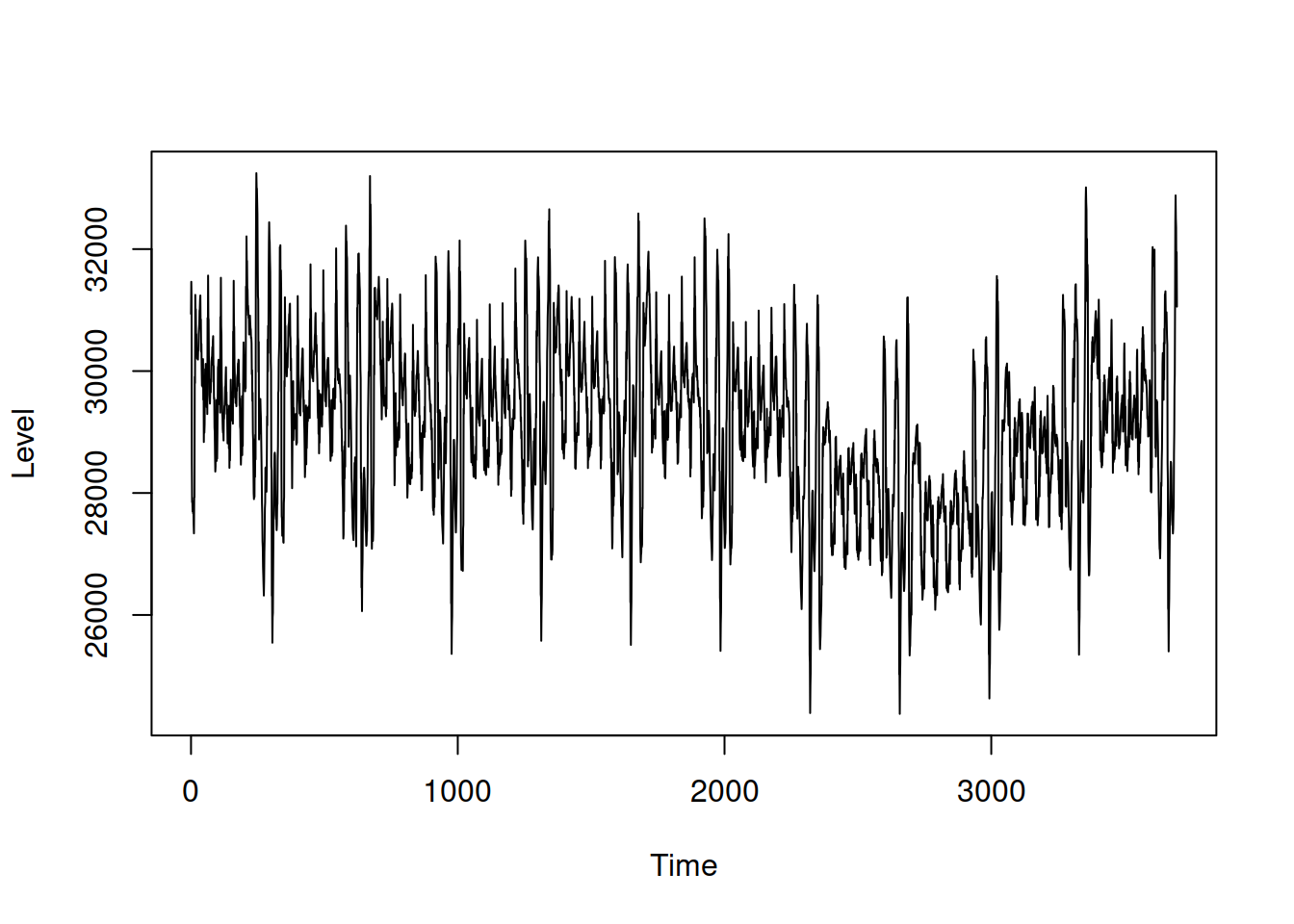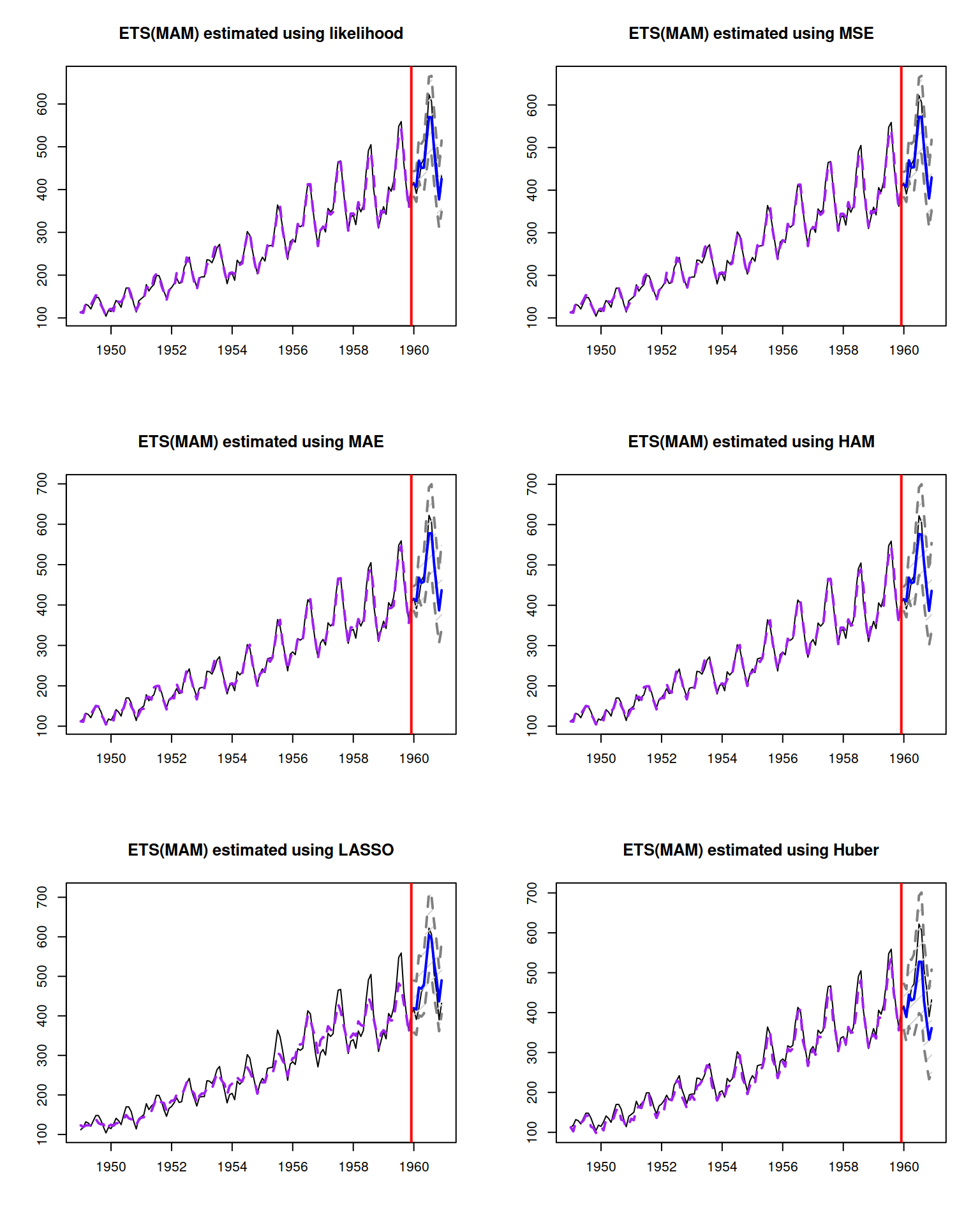This book is in Open Review. I want your feedback to make the book better for you and other readers. To add your annotation, select some text and then click the on the pop-up menu. To see the annotations of others, click the button in the upper right hand corner of the page

## 10.5 Examples in R

Building upon the example with AirPassengers data from the previous section, we will construct multiplicative ARIMA models and see, which one of them is the most appropriate for the data. As a reminder, the best additive ARIMA model was ARIMA(0,2,2)(0,2,2)$$_{12}$$, which had AICc of 1046.426. We will do something similar here, but using Log Normal distribution, thus working with logARIMA. In order to understand what model can be used in this case, we can take logarithm of data and see whaat happens with the components of time series:

plot(log(AirPassengers))We still have the trend in the data and the seasonality now corresponds to the additive one rather than the multiplicative (as expected). While we might still need the second differences for the non-seasonal part of the model, taking first differences for the seasonal should suffice. So we can test several models with different options for ARIMA orders:

adamModelLogSARIMA <- vector("list",3)
adamModelLogSARIMA[] <- adam(AirPassengers, "NNN", lags=c(1,12),
orders=list(ar=c(0,0), i=c(1,1), ma=c(1,1)),
h=12, holdout=TRUE, distribution="dlnorm")
adamModelLogSARIMA[] <- adam(AirPassengers, "NNN", lags=c(1,12),
orders=list(ar=c(0,0), i=c(2,1), ma=c(2,2)),
h=12, holdout=TRUE, distribution="dlnorm")
adamModelLogSARIMA[] <- adam(AirPassengers, "NNN", lags=c(1,12),
orders=list(ar=c(1,0), i=c(1,1), ma=c(2,1)),
h=12, holdout=TRUE, distribution="dlnorm")
names(adamModelLogSARIMA) <- c("logSARIMA(0,1,1)(0,1,1)",
"logSARIMA(0,2,2)(0,1,1)",
"logSARIMA(1,1,2)(0,1,1)")

The thing that is different between the models is the non-seasonal part. Using the connection with ETS, the first model should work on local level data, the second should be optimal for the local trend series and the third one is placed somewhere in between the two. We can compare the models based on AICc:

sapply(adamModelLogSARIMA, AICc)
## logSARIMA(0,1,1)(0,1,1) logSARIMA(0,2,2)(0,1,1)
##                    994.5576                   1104.4386
## logSARIMA(1,1,2)(0,1,1)
##                   1022.6285

It looks like the logARIMA(0,1,1)(0,1,1)$$_{12}$$ is more appropriate for the data. In order to make sure that we did not miss anything, we analyse the residuals of this model:

par(mfcol=c(2,1))
plot(adamModelLogSARIMA[],10:11)We can see that there are no significant coefficient on either ACF or PACF, so there is nothing else to improve in this model. We can then produce forecast from the model and see how it performed on the holdout sample:

adamModelLogSARIMA[]
## Time elapsed: 0.39 seconds
## Model estimated using adam() function: SARIMA(0,1,1)(0,1,1)
## Distribution assumed in the model: Log Normal
## Loss function type: likelihood; Loss function value: 478.9136
## ARMA parameters of the model:
## MA:
##  theta1 theta1
##    -0.2357    -0.4972
##
## Sample size: 132
## Number of estimated parameters: 16
## Number of degrees of freedom: 116
## Information criteria:
##       AIC      AICc       BIC      BICc
##  989.8271  994.5576 1035.9520 1047.5009
##
## Forecast errors:
## ME: -14.723; MAE: 15.617; RMSE: 20.464
## sCE: -67.309%; sMAE: 5.949%; sMSE: 0.608%
## MASE: 0.648; RMSSE: 0.653; rMAE: 0.205; rRMSE: 0.199
plot(forecast(adamModelLogSARIMA[],h=12,interval="prediction"))The ETS model closest to the logARIMA(0,1,1)(0,1,1)$$_{12}$$ would probably be ETS(M,M,M):

adamModelETS <- adam(AirPassengers, "MMM", h=12, holdout=TRUE)
adamModelETS
## Time elapsed: 0.16 seconds
## Model estimated using adam() function: ETS(MMM)
## Distribution assumed in the model: Inverse Gaussian
## Loss function type: likelihood; Loss function value: 468.5996
## Persistence vector g:
##  alpha   beta  gamma
## 0.7723 0.0176 0.0001
##
## Sample size: 132
## Number of estimated parameters: 17
## Number of degrees of freedom: 115
## Information criteria:
##       AIC      AICc       BIC      BICc
##  971.1992  976.5676 1020.2068 1033.3133
##
## Forecast errors:
## ME: -4.422; MAE: 15.626; RMSE: 21.726
## sCE: -20.217%; sMAE: 5.953%; sMSE: 0.685%
## MASE: 0.649; RMSSE: 0.693; rMAE: 0.206; rRMSE: 0.211

Comparing information criteria, ETS(M,M,M) should be preferred to logARIMA, but in terms of accuracy on the holdout, logARIMA is more accurate than ETS on this data.